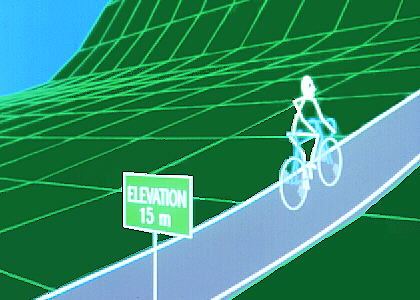# Derivatives

## Program 103The function of mathematics in physical science. From a theoretical concept to a practical tool, the derivative helps to determine the instantaneous speed and acceleration of a falling body. Differentiation is developed further to calculate how any quantity changes in relation to another. The power rule, the product rule, the chain rule -- with a few simple rules, differentiating any function becomes a simple mathematical task.